# Equation of motion for the translation of a single rod

• Engineering
Homework Statement:
The angular velocity of rod CD is at the moment shown in the figure 6 rad/s. A moment is applied at rod CD of M = 450 Nm. Determine the force in rod AB, the horizontal and vertical force at pin D and the angular acceleration in rod CD given at the moment shown in the figure. The block has a mass of 50 kg and his center of mass is at point G. Neglect the masses of the rods.
Relevant Equations:
(ag)n = w^2 * r
(ag)t = alpha * r
Hello,

Given the statement a described above. To find the forces at point D I drawn a kinematic scheme and FBD of rod CD. But why am I allowed to ignore the mass of 50 kg, the forces at point B and point A? I know the are some rules about this, but I just can't remember them anymore.. The figure of the situation can be found below.

See my sketch with calculations: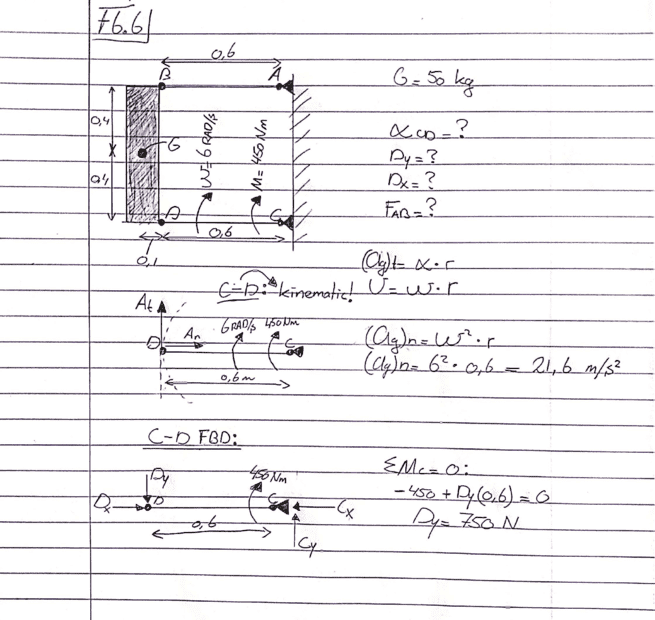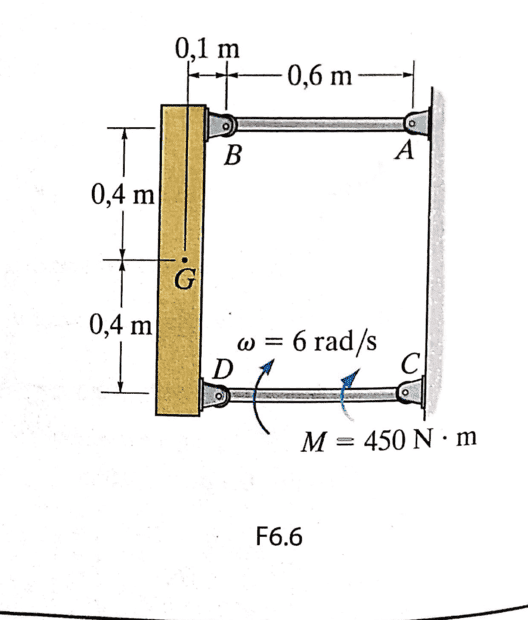Lnewqban
Gold Member
Why ignoring the mass of 50 Kg?
Acceleration of a massless system, under the action of a force, should be infinite.

Acceleration of a massless system, under the action of a force, should be infinite
But the system isn't massless? It moves/accelerates the 50 kg isn't it?

And the forces in A/B should only be considered in the total FBD?

Lnewqban
Gold Member
Links AB and CD do not have a mass value; their only function is to force point G, which represents a mass of 50 kg, to describe a semicircular movement that is pretty close to the trajectories of points B and D.
Link CD is under the effect of a torque or moment about pivot C, which induces the acceleration of those 50 Kg.
The centripetal component of that acceleration, combined with that mass, induces centripetal forces that are equally shared by links AB and CD of similar leghts (both points, B and D have same radius of rotation).

Last edited:
I am still a bit confused, what do you mean with centripetal component?

induces centripetal forces that are equally shared by links AB and CD of similar leghts
Do you mean that when the links AB and CD weren't the same length, the mass should be considered?

It is hard to imagine that point D only needs to overcome the torque while there is a mass pushing downwards with 50 kg on it.

Lnewqban
Gold Member
The mass should always be considered.
If the center of mass of that mass is rotating about a point away from it, there is a centripetal acceleration forcing it to do so.

The mass should always be considered.
But why does it kind of 'disappear' in this Sum of moment around point C? The force is pointed towards point C, but is still has a velocity which affects the moment around C?

which induces the acceleration of those 50 Kg.
I think this makes sense to me, after re-reading the topic for multiple times.

The mass should have been considered if there wasn't applied a moment around C. So if I erase that moment the mass should be considered? Otherwise the mass would drop downwards.

Lnewqban
Gold Member
I think this makes sense to me, after re-reading the topic for multiple times.

The mass should have been considered if there wasn't applied a moment around C. So if I erase that moment the mass should be considered? Otherwise the mass would drop downwards.
In what direction the mass will move depends on how strong the applied moment is.

In what direction the mass will move depends on how strong the applied moment is.
The mass direction is opposite to the moment, so downwards, right?

Lnewqban
Gold Member
The moment becomes a force at point D.
The mass, combined with gravity accelerataion, becomes another force.

Is this what you mean?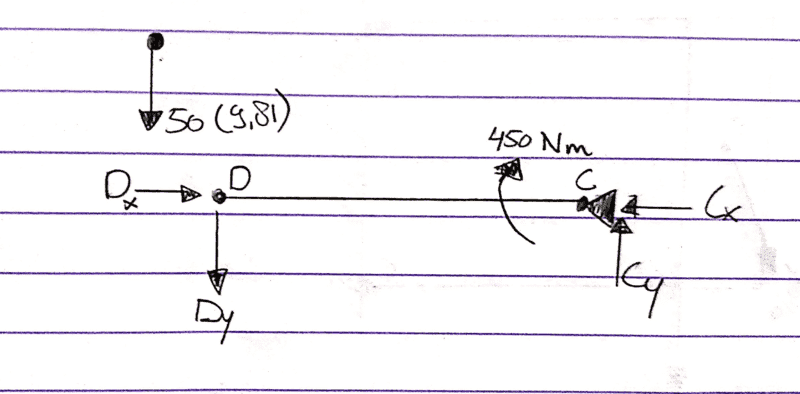Are those the FBD's for this structure?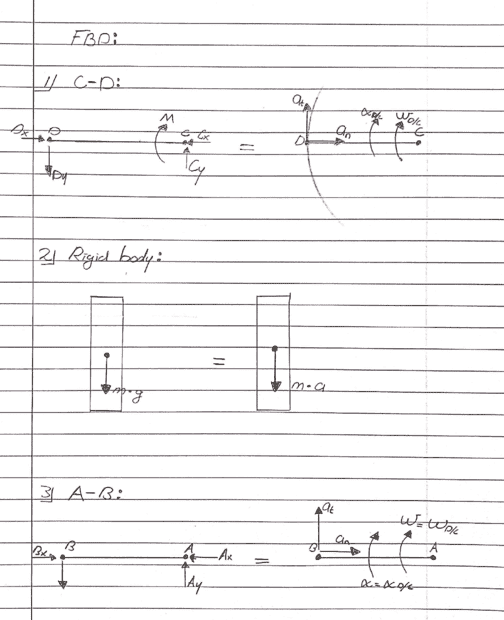Lnewqban
Gold Member
We have two vertical forces acting on D: M/0.6(upwards) and 50g (downwards).
Therefore, there is a resultant force pushing and accelerating G up at the instant represented in the diagram.

That makes the following (below) FBD?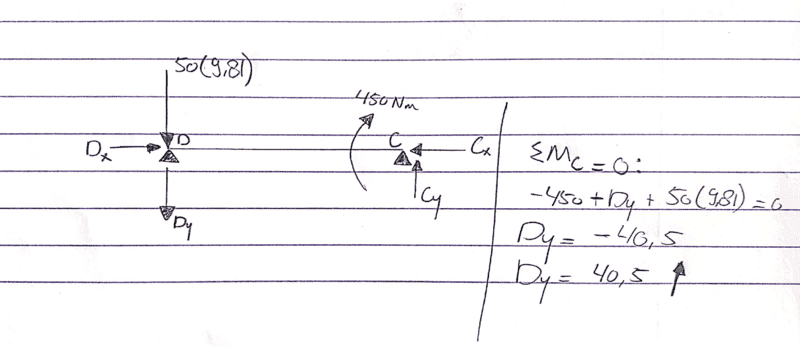Make that last FBD sense to you @Lnewqban ?

Like to hear :)

Anyone who could help?

Thanks